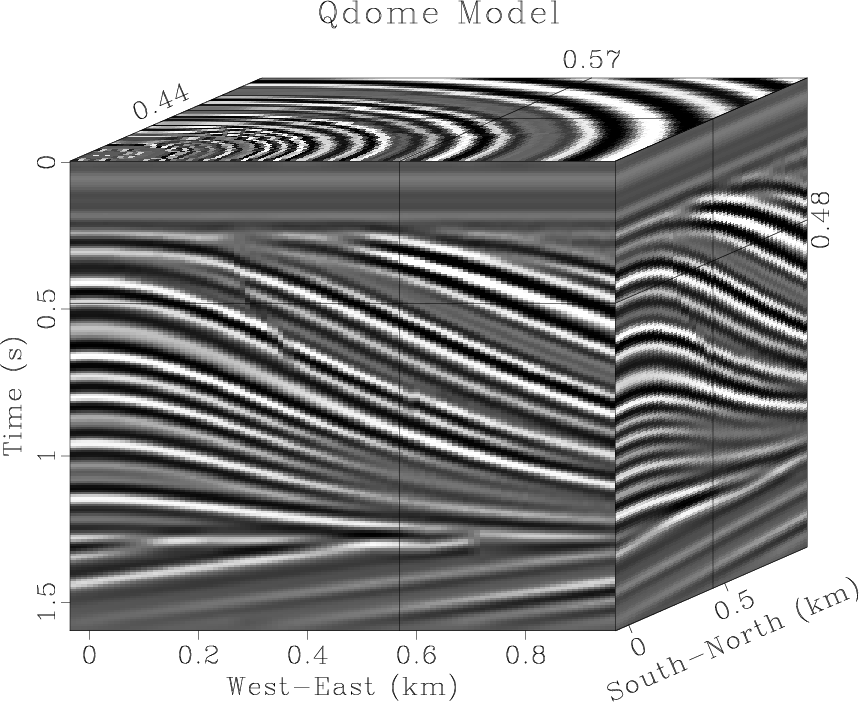### Month: April 2012

April 22, 2012 Celebration No comments

The 1.3 stable release features 7 new reproducible papers, multiple bug fixes, and a tutorial by Jeff Godwin.

According to the SourceForge statistics, the previous 1.2 stable release has been downloaded nearly 4,000 times, with more than 1,000 downloads in China. During the same period, there have been 41,028 read transactions and 994 write transactions in the Subversion repository. Ohloh.net characterizes Madagascar by a “mature, well-established codebase” and a “large, active development team“, the estimated project cost is \$7,4 million.## Program of the month: sfgrey3

April 1, 2012 Programs No comments

sfgrey3 generates “cube” plots from 3-D data. A cube plot displays sections from a 3-D cube as faces of the cube.

Some of the options specific to this kind of display are:

• flat= The flat parameter tells sfgrey3 whether to display the cube as a flat image or in a 3-D projection. The default is flat=y. The following example from bei/fld/cube displays the same input data with both options:• frame1=, frame2=, frame3= The frame parameters select the slices from the cube to be displayed in the plot. The default is to take the first slice from the vertical and the out-of-plane axis (frame1=0 frame3=0) and the last slice from the horizontal axis (frame2=n2-1). In the following example from geo384w/hw3/gulf, the data size is n1=1000 n2=250 n3=48 and the frame parameters are frame1=500 frame2=160 frame3=10:• point1=, point2= The point1= and point2= parameters control the aspect ratio of the cube by specifying the ratio (from 0 and 1) that the front face of the cube takes from the plot in the vertical and the horizontal dimension, respectively. The default is point1=0.5 point2=0.5. The following example from sep/plane/qint has point1=0.85 point2=0.74:• movie= The movie parameter allows the user to create a movie looping over slices in a cube instead of a static picture. The value of the parameter (from 1 to 3) indicates the axis for looping or 0 (the default) for no movie. The frame increment in the movie is controled by dframe=. The following example from cwp/geo2009TTIModeSeparation/tti3 uses movie=1 to loop over the vertical axis:To generate a movie out of 4-D data, you can use sfgrey4. Here is an example: